• 频率响应函数、单位脉冲响应函数、单位脉冲响应函数与频率响应函数的关系、卷积定理。
• 以信息通道的写读脉冲响应概念为基础，建立了光盘数据存储系统的模型，得到了脉冲响应函数和频率传递函数，分析了光学头的伺服误差和像差对读出过程的影响．
• 建模以检查脉冲响应函数的 MATLAB 代码 主要代码称为 TEMPLATE。 其他文件是在主代码中调用的函数。 代码是为 Matlab 编写的，应该很容易转换为 Python（使用 python 包进行协整） 第 1 部分使用 txt 文件设置数据。...
• 探讨减少非线性系统广义脉冲响应函数( GIRF) 盲辨识所需计算量问题。基于线性 MIMO 模 型, 应用多项式矩阵理论和子空间盲辨识技术,研究使用部分噪声向量对非线性 Vo lter r a系统的 GIRF 盲辨识方法。该...
• 运用基于VAR模型的脉冲响应函数,对我国西部地区1992―2011年外国直接投资流入(IFDI)和进出口贸易之间的长期动态关系进行了动态研究。脉冲响应函数分析结果表明,IFDI对中国西部地区的出口贸易呈负效应,而对中国西部...
• 明确地给出了刚性边界条件下房间内两点之间声传输函数和声脉冲响应函数的有限元素法计算模型,并以矩形房间为例详细讨论了有关细节。所讨论的计算模型可以真实地反映房间内不同声源点、接收点位置上的声压频谱特性和...
• 梯度系统的梯度脉冲响应函数 (GIRF) 的代码包。 GIRF 计算和轨迹预测的基本功能在 test 文件夹中的“DemoGirf.m”中进行了演示。 确保文件夹“classes”和“utils”位于 Matlab 路径上。 如果您使用此代码包进行研究...
• 结合蒙特卡罗均值算法和广义脉冲响应函数模型，构造一个用于分析含多个外生变量的向量自回归模型的新算法．将该算法应用于我国货币政策的非对称性效应分析中，以实验结论证明算法的可行性和有效性．
• 经典的相关系数法求解系统的脉冲响应函数 准确率非常高。
• 对于受白噪声激励的系统，使用自然激励技术和时域方法 (NExTT) 和频域方法 (NExTF) 返回脉冲响应函数 (IRF)。 提供了示例文件，用于估计受到高斯白噪声激励的 2DOF 系统的 IRF，并为响应增加了不确定性（也是高斯白...matlab
• 本文通过建立VAR模型，通过脉冲响应函数与方差分解的方法，研究了GDP与居民可支配收入变化冲击对房地产价格的动态影响．实证结论表明：GDP变化所带来的冲击会给房地产价格带来一个长期的正向影响，短期内房地产价格...
• 用于评估分数阶传递函数脉冲响应的工具箱。 有两种计算脉冲响应的方法。 一种方法基于 Mittag-Leffler 函数，第二种方法基于广义拉盖尔函数。 致谢： 该工具箱是在作者访问斯洛伐克科希策技术大学 BERG 学院生产...matlab
• ## 使用Stata做脉冲响应分析

万次阅读 多人点赞 2019-04-25 21:20:42
在这篇推文中，我们讨论 VAR 模型中的脉冲响应函数(IRFs)。 脉冲响应函数反映了当 VAR 模型某个变量受到"外生冲击"时，模型中其他变量受到的动态影响。我们会根据这些变量受到此冲击后的一段时间内的动态变化画出...
Source: Rizaudin Sahlan → Impulse Response Function with Stata (time series)
在这篇推文中，我们讨论 VAR 模型中的脉冲响应函数(IRFs)。
脉冲响应函数反映了当 VAR 模型某个变量受到"外生冲击"时，模型中其他变量受到的动态影响。我们会根据这些变量受到此冲击后的一段时间内的动态变化画出脉冲响应图形。
脉冲响应函数是一种条件预测，更确切地说，是一种点估计，只不过我们会估计冲击发生后不同时点的值。
类似于 AR 模型有 MA 表达形式，VAR 模型也有 VMA 表达形式。 VMA 表达形式有助于我们探求在 VAR 系统中变量收到冲击后的随时间变化的路径。考虑一个由 $y_t$ 和 $z_t$ 构成的VAR 系统：
$\left[ \begin{array} { c } { y _ { t } } \\ { z _ { t } } \end{array} \right] = \left[ \begin{array} { c } { a _ { 10 } } \\ { a _ { 20 } } \end{array} \right] + \left[ \begin{array} { c c } { a _ { 11 } } & { a _ { 12 } } \\ { a _ { 21 } } & { a _ { 22 } } \end{array} \right] \left[ \begin{array} { c } { y _ { t - 1 } } \\ { z _ { t - 1 } } \end{array} \right] + \left[ \begin{array} { c } { e _ { 1 t } } \\ { e _ { 2 t } } \end{array} \right]\tag{1}$
该模型也可以写成如下形式：
$\left[ \begin{array} { c } { y _ { t } } \\ { z _ { t } } \end{array} \right] = \left[ \begin{array} { c } { \overline { y } } \\ { \overline { z } } \end{array} \right] + \sum _ { i = 0 } ^ { \infty } \left[ \begin{array} { c c } { a _ { 11 } } & { a _ { 12 } } \\ { a _ { 21 } } & { a _ { 22 } } \end{array} \right] ^ { i } \left[ \begin{array} { c } { e _ { 1 t - i } } \\ { e _ { 2 t - i } } \end{array} \right] \tag{2}$
方程 (2) 使用 $\left\{ e_ { 1 t } \right\}$ 和 $\left\{ e_ { 2 t } \right\}$ 序列表示了 $y_t$ 和 $z_t$ 。根据 Enders(2014, p286)， $\left\{ e_ { 1 t } \right\}$ 和 $\left\{ e_ { 2 t } \right\}$ 可以写成：
$\left[ \begin{array} { c } { e _ { 1 t } } \\ { e _ { 2 t } } \end{array} \right] = \frac{1}{ 1 - b _ { 12 } b _ { 21 }} \left[ \begin{array} { c c } { 1 } & { - b _ { 12 } } \\ { - b _ { 21 } } & { 1 } \end{array} \right] \left[ \begin{array} { c } { \varepsilon _ { y t } } \\ { \varepsilon _ { zt } } \end{array} \right]\tag{3}$
将 (3) 式代入 (2) 式中可得：
$\left[ \begin{array} { c } { y _ { t } } \\ { z _ { t } } \end{array} \right] = \left[ \begin{array} { c } { \overline { y } } \\ { \overline { z } } \end{array} \right]+ \frac{1}{ 1 - b _ { 12 } b _ { 21 }} \sum _ { i = 0 } ^ { \infty } \left[ \begin{array} { c c } { a _ { 11 } } & { a _ { 12 } } \\ { a _ { 21 } } & { a _ { 22 } } \end{array} \right] ^ { i } \left[ \begin{array} { c c } { 1 } & { - b _ { 12 } } \\ { - b _ { 21 } } & { 1 } \end{array} \right] \left[ \begin{array} { c } { \varepsilon _ { y t } } \\ { \varepsilon _ { z t } } \end{array} \right] \tag { 4 }$
由于 (4) 式不太简洁，我们可以通过定义一个 $2\times2$ 的矩阵 $\phi_i$ 来简化它：
$\phi _ { i } = \frac{A _ { 1 } ^ { i } }{ 1 - b _ { 12 } b _ { 21 } } \left[ \begin{array} { c c } { 1 } & { - b _ { 12 } } \\ { - b _ { 21 } } & { 1 } \end{array} \right]\tag{5}$
将 (5) 式代入 (4) 式可得：
$\left[ \begin{array} { l } { y _ { t } } \\ { z _ { t } } \end{array} \right] = \left[ \begin{array} { c } { \overline { y } } \\ { \overline { z } } \end{array} \right] + \sum _ { i = 0 } ^ { \infty } \left[ \begin{array} { c c } { \phi _ { 11 } ( i ) } & { \phi _ { 12 } ( i ) } \\ { \phi _ { 21 } ( i ) } & { \phi _ { 22 } ( i ) } \end{array} \right] ^ { i } \left[ \begin{array} { c } { \varepsilon _ { y t - i } } \\ { \varepsilon _ { zt - i } } \end{array} \right]\tag{6}$
显然 (6) 式可以写成更简洁的形式：
$x _ { t } = \mu + \sum _ { i = 0 } ^ { \infty } \phi _ { i } \varepsilon _ { t - i }\tag{7}$
以上 VMA 形式的表出对于我们理解  $y_t$ 和 $z_t$ 构成的VAR 系统格外有帮助。其中系数矩阵 $\phi_i$ 即为来自 $\varepsilon_{yt}$ 和  $\varepsilon_{zt}$ 的冲击对 $y_t$ 和 $z_t$ 序列的影响。
很显然 $\phi_{jk} (0)$ 是冲击的即时影响。比如，$\phi_{12} (0)$ 表示 1 个单位  $\varepsilon_{zt}$ 的冲击会使当期的  $y_t$ 变化多少个单位。类似地，$\phi_{11} (1)$ 和 $\phi_{12} (1)$ 则分别表示来自一个单位  $\varepsilon_{yt-1}$ 和一个单位   $\varepsilon_{zt-1}$ 的冲击对  $y_t$ 产生的影响。
显然 $\phi_{11} (1)$ 和 $\phi_{12} (1)$ 也可以表示来自一个单位  $\varepsilon_{yt}$ 和  $\varepsilon_{zt}$ 的冲击对  $y_{t+1}$ 产生的影响。以此类推，来自一个单位  $\varepsilon_{yt}$ 和  $\varepsilon_{zt}$ 的冲击对后续各期的  $y_t$ 和 $z_t$ 产生的影响可以通过对脉冲响应函数进行适当的汇总得到。比如来自一个单位  $\varepsilon_{zt}$ 的冲击对 {$y_{t}$} 序列在后续第 $n$ 期产生的影响即为 $\phi_{12} (n)$ ，则在此  $\varepsilon_{zt}$ 冲击对 $y_{t}$ 序列在冲击发生后的 $n$ 期内产生的总影响为：
$\sum _ { i = 0 } ^ { n } \phi _ { 12 } ( n )$
令 $n$ 趋于无穷，可以得到冲击对  $y_t$ 和 $z_t$ 产生的长期影响。由于 {$y_{t}$} 和 {$z_{t}$} 被假定为平稳的，易得对于任意 $j$  和 $k$ ：
$\sum _ { i = 0 } ^ { \infty } \phi _ { j k } ^ { 2 } ( i )\text{ 在 i\rightarrow \infty 时收敛}$
四个系数集 $\phi_ { 11 } ( i )$，$\phi _ { 12 } ( i )$，$\phi _ { 21 } ( i )$ 和 $\phi _ { 22 } ( i )$ 被称为脉冲响应函数。绘制脉冲响应函数的图形是观察冲击  $\varepsilon_{yt}$ 和  $\varepsilon_{zt}$ 对系统内变量  $y_t$ 和 $z_t$ 影响的实用手段，也是观察来自  $\varepsilon_{yt}$ 和  $\varepsilon_{zt}$ 冲击造成的时变影响的有力工具。
使用 Stata 估计脉冲响应函数
在 Stata 中， 我们可以使用 irf create 命令得到脉冲响应函数，这个命令可以估计五种脉冲响应函数(IRFs)：简单脉冲响应函数(simple IRFs)、正交脉冲响应函数(orthogonalized IRFs)、累积脉冲响应函数(cumulative IRFs)、累积正交脉冲响应函数(cumulative orthogonalized IRFs)以及结构脉冲响应函数(structural IRFs)。
具体的操作思路为：首先拟合 VAR 模型，然后使用 irf create 命令估计脉冲响应函数并将其存储到文件中，最后使用 irf graph 或其他的 irf 分析命令检验结果。
本篇推文使用的数据集为 Data09.dta，可以点击这里下载。
我们想估计的 VAR 模型中包含的变量有：对数固定资本形成总额 (lrgrossinv) ，对数实际家庭消费支出额 (lrconsump) 以及对数 GDP (lrgdp)。
在估计脉冲响应函数之前，我们首先需要知道该 VAR 模型的最优滞后阶数：
 varsoc lrgrossinv lrconsump lrgdp, max (12)

Selection-order criteria
Sample:  1962q1 - 2010q3                     Number of obs      =       195
+---------------------------------------------------------------------------+
|lag |    LL      LR      df    p      FPE       AIC      HQIC      SBIC    |
|----+----------------------------------------------------------------------|
|  0 |  721.692                      1.3e-07   -7.3712  -7.35081  -7.32085  |
|  1 |  1979.02  2514.7    9  0.000  3.5e-13  -20.1746   -20.093  -19.9731  |
|  2 |  2022.28  86.525    9  0.000  2.4e-13   -20.526  -20.3833* -20.1735* |
|  3 |  2030.07  15.571    9  0.076  2.5e-13  -20.5135  -20.3096    -20.01  |
|  4 |  2036.31  12.492    9  0.187  2.5e-13  -20.4853  -20.2202  -19.8307  |
|  5 |  2044.65  16.669    9  0.054  2.6e-13  -20.4784  -20.1522  -19.6728  |
|  6 |  2056.46  23.622    9  0.005  2.5e-13  -20.5073  -20.1199  -19.5505  |
|  7 |  2070.89  28.858*   9  0.001  2.4e-13*  -20.563* -20.1144  -19.4552  |
|  8 |  2074.97  8.1629    9  0.518  2.5e-13  -20.5125  -20.0028  -19.2537  |
|  9 |  2078.94  7.9406    9  0.540  2.6e-13  -20.4609  -19.8901   -19.051  |
| 10 |  2083.77  9.6582    9  0.379  2.7e-13  -20.4181  -19.7861  -18.8572  |
| 11 |  2088.52  9.5076    9  0.392  2.9e-13  -20.3746  -19.6814  -18.6626  |
| 12 |     2094  10.959    9  0.279  3.0e-13  -20.3385  -19.5841  -18.4754  |
+---------------------------------------------------------------------------+
Endogenous:  lrgrossinv lrconsump lrgdp
Exogenous:  _cons

以上结果显示，根据 AIC 准则，模型的最优滞后结束为 7 阶。使用 7 阶滞后重新估计 VAR 模型：
quietly var lrgrossinv lrconsump lrgdp,lags(1/7)dfk small

并基于估计结果估计脉冲响应函数：
 irf create order1, step(10) set(myirf1) replace
(file myirf1.irf created)
(file myirf1.irf now active)
irfname order1 not found in myirf1.irf
(file myirf1.irf updated)

在 Stata 中，多个脉冲响应的结果可以被存储在同一个文件中，并被标记为不同的名字。上述命令表示，此次脉冲响应估计的结果被存储在 myirf1 文件中，其名字被标记为 order1。order1 中存储了前面提到的该 VAR 模型的全部五种脉冲响应函数的估计结果。
得到了脉冲响应函数的估计结果后，我么就可以使用 irf graph 命令来绘制秒冲响应图形了：
irf graph oirf, impulse(lrgrossinv lrconsump lrgdp) response(lrgrossinv lrconsump lrgdp)  yline (0,lcolor(black)) byopts(yrescale)脉冲响应图形中，每一行为同种冲击对不同变量造成的影响，每一列为不同冲击对同一变量造成的影响。横坐标的刻度单位为 VAR 模型估计的单位时间(在这个案例中为每一季度)。这张图形中显示的是冲击在 10 个季度内造成的影响。纵坐标以每个变量自己的单位来衡量，由于我们估计的模型所有变量的单位均为百分比，因此纵坐标表示百分比的变化。
第一行展示了家庭消费 (lrconsump) 受到一个单位标准差的冲击对 VAR 系统造成的影响：家庭消费 (lrconsump) 会瞬间全部吸收冲击，并增加相应单位的百分比，此影响甚至到 10 个季度之后都没有消退。GDP (lrgdp) 受到该冲击的影响会在4个季度内有所上升，在第四个季度达到峰值，随后该影响慢慢衰退。固定资本形成额 (lrconsump) 与 GDP 呈现相似的变化。
第二行展示了GDP (lrgdp) 受到一个单位标准差的冲击对 VAR 系统造成的影响：家庭消费 (lrconsump) 和固定资本形成额 (lrconsump) 都在前 5 个季度轻微下降，之后回升。
第三行展示了固定资本形成额 (lrconsump) 受到一个标准差的冲击对 VAR 系统造成的影响：家庭消费 (lrconsump) 和 GDP (lrgdp) 都出现了高度持续的下降。


展开全文时间序列 VAR
• 基于脉冲响应辨识的matlab程序，对过程施加M序列的扰动，辨识起过程脉冲响应函数
• matlab开发-脉冲响应方差双字节解切断传递函数的描述。BICO传递函数脉冲响应不变离散化Simulink基础
• 基于已知的输入 (u) 和输出 (y)，该函数返回脉冲响应的系数以及原始信号与重建信号的信噪比（以 dB 为单位）。 鼓励用户尝试各种响应长度并保留最短的响应长度，从而产生可接受的噪声信号比。 参考：Karel J. ...matlab
• 摘要：这是一个应用举例，A=【1 -1.5 0.7】，B=【1 0.5】，nk=1 ， e为噪声项，分为三种情况，在这三种情况下，采用伪随机信号作为输入，应用相关分析法辨识系统的脉冲响应函数，并辨识出系统的模型参数。...matlab
• 本文以上海市为实证对象, 通过构建政策变量对经济效果变量的脉冲响应函数模型, 揭示了国家重大区域规划政策对上海市经济发展的影响. 通过构建政策变量对经济效果变量的...
• 目录 模型与数据 估算值 预测误差脉冲响应 ...脉冲响应分析是采用向量自回归模型的计量经济学分析中的重要一步。它们的主要目的是描述模型变量对一个或多...这篇文章介绍了VAR文献中常用的脉冲响应函数的概念和解...
原文链接：http://tecdat.cn/?p=9384

原文出处：拓端数据部落公众号

目录

模型与数据

估算值

预测误差脉冲响应

识别问题

正交脉冲响应

结构脉冲反应

广义脉冲响应

参考文献

脉冲响应分析是采用向量自回归模型的计量经济学分析中的重要一步。它们的主要目的是描述模型变量对一个或多个变量的冲击的演化。因此使它们成为评估经济时非常有用的工具。这篇文章介绍了VAR文献中常用的脉冲响应函数的概念和解释。

模型与数据

为了说明脉冲响应函数的概念，使用了Lütkepohl（2007）的示例。可以从教科书的网站上下载所需的数据集。它包含从1960年1季度到1982年4季度按季度和季节性调整的时间序列，这些序列是西德的固定投资，可支配收入和数十亿德国马克的消费支出。

# 下载数据
data <- read.table("e1.dat", skip = 6, header = TRUE)

# 仅使用前76个观测值，因此有73个观测值
# 取一阶差分后，留给估计的VAR(2)模型。
data <- data[1:76, ]

# 转换为时间序列对象
data <- ts(data, start = c(1960, 1), frequency = 4)

# 取对数和差值
data <- diff(log(data))

# 绘图数据
plot(data,  main = "Dataset E1 from Lütkepohl (2007)")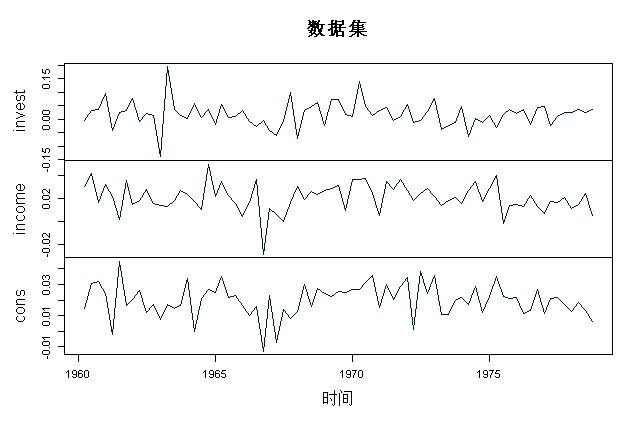此数据用于估计具有常数项的VAR（2）模型。

估算值

可以使用vars软件包估算VAR模型：


# 查看摘要统计信息
summary(model)

代码的结果应与Lütkepohl（2007）的3.2.3节中的结果相同。

预测误差脉冲响应

由于VAR模型中的所有变量都相互依赖，因此单独的系数估计仅提供有关反应的有限信息。为了更好地了解模型的动态行为，使用了脉冲响应（IR）。线性VAR模型的每个脉冲响应函数的出发点都是其移动平均值（MA）表示，这也是预测误差脉冲响应（FEIR）函数。

在R 中，程序包可用于获取预测误差脉冲响应。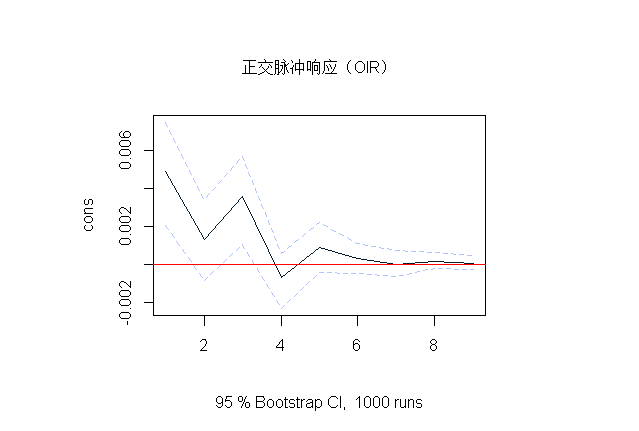识别问题

从上图可以看出，在第一期间FEIR为零。对于使用的数据集，估计为

##              invest       income         cons
## invest 2.129629e-03 7.161667e-05 1.232404e-04
## income 7.161667e-05 1.373377e-04 6.145867e-05
## cons   1.232404e-04 6.145867e-05 8.920351e-05

由于估计方差-协方差矩阵的非对角线元素不为零，因此我们可以假设VAR模型中的变量之间存在同期相关性。这由与Σ相对应的相关矩阵确认：

##           invest    income      cons
## invest 1.0000000 0.1324242 0.2827548
## income 0.1324242 1.0000000 0.5552611
## cons   0.2827548 0.5552611 1.0000000

但是，这些矩阵仅描述了误差之间的相关性，但不清楚因果关系的方向。识别这些因果关系是任何VAR分析的主要挑战之一。

正交脉冲响应

识别VAR模型的冲击的常用方法是使用正交脉冲响应（OIR）。基本思想是分解方差-协方差矩阵，使∑ = PP− 1，其中P是带有正对角线元素的下三角矩阵，通常通过Choleski分解获得。给定估计方差-协方差矩阵PP，可以通过以下方法获得分解


##             invest      income        cons
## invest 0.046147903 0.000000000 0.000000000
## income 0.001551894 0.011615909 0.000000000
## cons   0.002670552 0.004934117 0.007597773


从这个矩阵可以看出，收入冲击对消费具有同时性的影响，反之则不然。

在R 中，vars可以通过设置参数来使用包的功能来获得OIR：


plot(oir)请注意，Choleski分解的输出是一个较低的三角矩阵，因此第一行中的变量永远不会对任何其他变量的同时冲击敏感，而系统中的最后一个变量将对所有其他变量的冲击敏感。因此，OIR的结果可能对变量的顺序很敏感，建议用不同的顺序估计上述VAR模型，以查看所产生的OIR受此影响的程度。

结构脉冲反应

在VAR模型的估计过程中，结构脉冲响应（SIR）已经考虑了识别问题。

广义脉冲响应

正交和结构响应都可以通过找到变量的正确顺序或通过识别估计的结构参数来约束。Koop等（1998）提出了一种不同类型的响应函数，即所谓的广义脉冲响应（GIR）。它们独立于变量顺序，因为它们将其他冲击的影响整合到响应之外。

对于难以识别结构关系的大型系统，GIR非常有用。


展开全文R语言 VAR模型
• MATLAB帮助文档对impz的介绍：【 MATLAB 】impz函数介绍（数字滤波器的脉冲响应） 我在 MATLAB 中查看 impz 的帮助文档时，始终看的不是太明白这个函数的使用，于是我根据一个例子，对这个函数做了一点分析，解决了...
这篇博文在于讨论 impz 函数的使用。

MATLAB帮助文档对impz的介绍：【 MATLAB 】impz函数介绍（数字滤波器的脉冲响应）

我在 MATLAB 中查看 impz 的帮助文档时，始终看的不是太明白这个函数的使用，于是我根据一个例子，对这个函数做了一点分析，解决了一些我的疑惑，记录于此。

帮助文档上对这个函数的概括是数字滤波器的脉冲响应。

所谓的数字滤波器不就是一个线性时不变系统而已，根据脉冲响应的分子分母系数，可以得到该系统的脉冲响应。这个脉冲响应可以用下面的有理传递函数给出：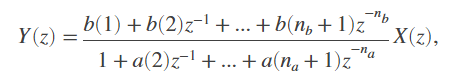或者由差分方程给出：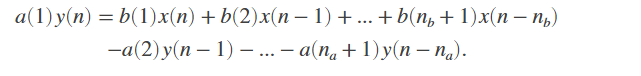上面的系数用系数向量表示为：

分母系数为：

分子系数为：

因此，impz函数的语法格式有这么一条：

[h,t] = impz(b,a)

这里的b和a向量就是分子和分母系数的向量。

得到的h就是该系统的脉冲响应，而 t 是脉冲响应值对应的位置。

对于这个函数的使用，可以直接使用右边的部分，而不出现左边的部分，即 impz(b,a)，而文档对其解释如下：

impz(...) with no output arguments plots the impulse response of the filter.

意思是直接画出该系统的脉冲响应。

我们举个例子来理解：

这个系统使用差分方程来描述如下：

y(n) - y(n-1) + 0.9y(n-2) = x(n)

要求计算并画出它的脉冲响应。

题解：

跟上上述对impz函数地简单介绍，其实我们可以直接画出它的脉冲响应了。

b = ;
a = [1,-1,0.9];
[h,t] = impz(b,a)
stem(t,h);
title('Impulse Response');
xlabel('n');ylabel('h(n)');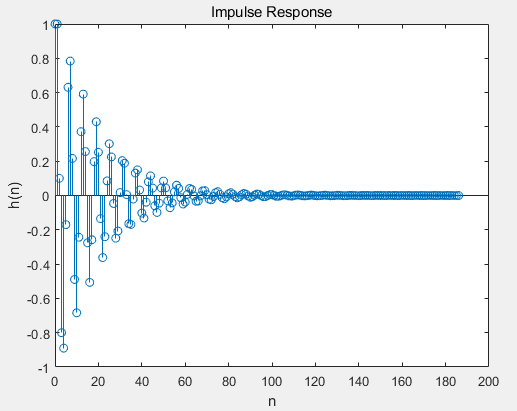也可以：

clc
clear
close all

b = ;
a = [1,-1,0.9];

impz(b,a);
ylim([-1.2,1.2]);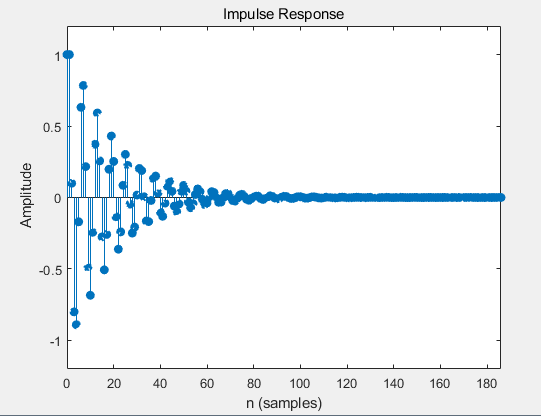上面两种方法画出的脉冲响应其实是一样的。

上面这个简单的用法也就告一段落，下面我们再看一条变体语法：

[h,t] = impz(...,n) computes n samples of the impulse response when n is an integer (t = [0:n-1]'). If n is a vector of integers, impz computes the impulse response at those integer locations, starting the response computation from 0 (and t = n or t = [0 n]). If, instead of n, you include the empty vector, [], for the second argument, the number of samples is computed automatically.
只看语法格式，那么一大串英文介绍暂时不管，后面我会慢慢解释。

[h,t] = impz(...,n)

后面的这个n是什么玩意？

它的用意我用自己的语言叙述下就是如果我要求的脉冲响应不是从0开始，那么我就可以使用这个n向量来指定脉冲响应的位置范围。

同样使用一个实例来说明，同样是上面的那个差分方程表示的系统，我们求它的脉冲响应。

这个系统使用差分方程来描述如下：

y(n) - y(n-1) + 0.9y(n-2) = x(n)

要求计算并画出在 的脉冲响应h(n)。

脚本程序如下：

clc
clear
close all

b = ;
a = [1,-1,0.9];
n = [-20:120]';

impz(b,a,n);
ylim([-1.2,1.2]);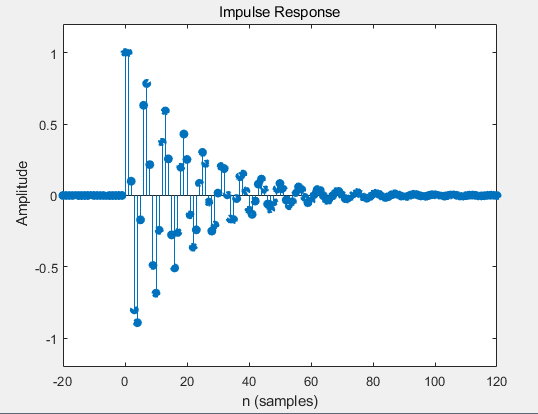这里的n是一个行向量或者一个列向量，无关紧要。

也可以使用下面的方法来产生：

clc
clear
close all

b = ;
a = [1,-1,0.9];
n = [-20:120]';

[h,t] = impz(b,a,n)
stem(t,h);
title('Impulse Response');
xlabel('n');ylabel('h(n)');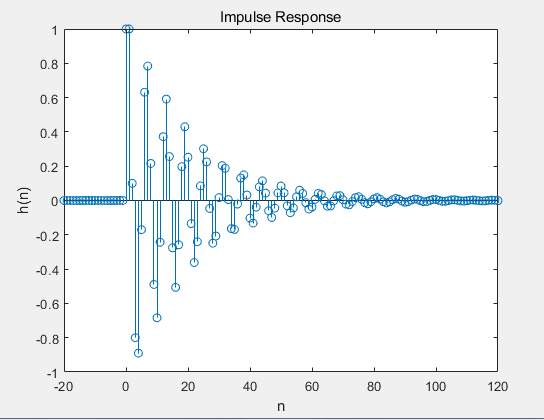上面画图的函数stem(t,h)，也可以改成stem(n,h)。

这间接说明了t和n是一样的，函数产生的t是一个列向量，如果n也是一个列向量的话，那么二者一致。

可以使用这条语句进行验证：

n == h

可以得到一个和n同维度的向量，元素全为1.（可自行验证。）

最后，需要说明的是由于是同一个系统，所以指定与不指定n产生的脉冲响应都是一样的，只不过这里指定了显示脉冲响应的位置在-20，...,100.

再给出一个例子：

首先使用ellip函数产生一个满足如下要求的滤波器，关于ellip函数介绍如下：

【 MATLAB 】ellip 函数介绍（椭圆滤波器设计）

设计具有归一化通带频率0.4的四阶低通椭圆滤波器。 指定0.5 dB的通带纹波和20 dB的阻带衰减。 绘制脉冲响应的前50个样本。

clc
clear
close all
%
% Impulse Response of an Elliptic Lowpass Filter
% Design a fourth-order lowpass elliptic filter with normalized passband frequency 0.4.
% Specify a passband ripple of 0.5 dB and a stopband attenuation of 20 dB.
% Plot the first 50 samples of the impulse response.
[b,a] = ellip(4,0.5,20,0.4);
impz(b,a,50)展开全文• % 计算对入射 KdV 孤子脉冲的搁架响应。 文章中提出的分析模型的修改版本：A Model for the Generation of Coastal Seiches 由深海内波。 J.物理。 Oceanogr.,20,1459-1467.doi: ...matlab
• 严格适合SISO系统的脉冲响应计算程序： */num = 传递函数的分子多项式系数*/den = 传递函数的分母多项式系数（“ num”和“ den”的系数被指定为行向量，在's' 的递减幂） */tO = 施加单位脉冲输入的时间*/dt = ...matlab
• 博客写作技巧： 遇到的问题-如何解决问题-需要那种帮助 信道脉冲响应：CIR 问题： ...对于LTI系统信道脉冲响应类似于一种函数的作用规则。 如何获取信道脉冲响应？ 传输函数 状态空间矩阵 传播模型与信道无线通信
• 为了研究冲击传递的过程，我们引入了脉冲响应函数的概念，该函数描述了冲击对通过动态 copula 模型建模并使用 Kendall tau 和尾部相关系数进行测量的联系的影响的时间分布。 这种方法用于分析在 2007-2009 年危机...
• 工业化进程导致的工业废水排放量与工业用水技术效率有关,为了量化研究工业产值、工业废水排放量与工业用水技术效率三者之间的关系,对数据检验之后,建立VAR模型,运用脉冲响应函数和方差分解方法进行分析.结果表明:...
• 函数计算任意尺寸矩形房间中任意位置声源的房间脉冲响应，因为它会在任意位置被听到。 RIR.m 的输出是一个向量，可用作 FIR 滤波器以产生混响效果。 要实现此过滤器，您需要进行快速卷积。 我有另一个程序 FCONV.m...matlab
• 讨论了带有 Beddington-DeAngelis功能响应函数脉冲 predator-prey系统 ,运用比较定理和 Lia-punov函数 ,给出了 Predator常数周期脉冲迁移环境下系统持久和 prey灭绝的条件。
• 3.2 基本信号与基本响应 第三章离散系统的时域分析 知识点Z3.16 Matlab求解单位脉冲响应 主要内容 Matlab求解单位脉冲响应函数 基本要求 掌握单位脉冲响应的Matlab求解方法 1 Xidian University, ICIE. All Rights...
• 已知传递函数，求其脉冲响应 code clear clc num=[0 1 3] den=[1 2 3] % num与den要相同长度 grid impulse(num,den) result   resource [文档] ww2.mathworks.cn/help/matlab [文档] ww2.......